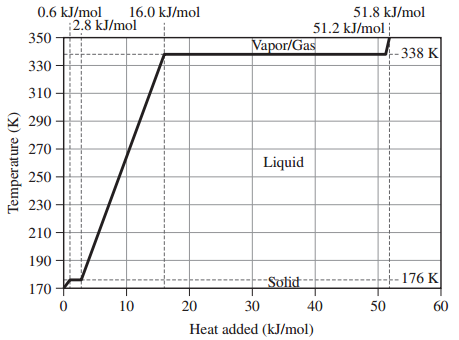# Problem: Assume that the values given here for 1 mole of methanol are constant over the relevant temperature ranges.Melting Point176 KBoiling Point338 KHfus2.2 kJ/mol KHvap35.2 kJ/mol KCs,solid105 J/mol KCs,liquid81.3 J/mol KCs,gas48 J/mol KGiven the heating curve for 1 mole of methanol beginning at 170 K and ending at 350 K answer the following questions:What is the value of qsolid for methanol?

###### FREE Expert Solution

Given the heating curve for 1 mole of methanol, we are asked to calculate qsolid for methanol.

Recall: In Heating curves, we have the representation of the amount of heat absorbed during phase changes. As the reaction progress, a phase change or temperature change can occur.

During a phase change,

• Temperature is constant
• Avg. Kinetic Energy is constant
• Heat is transferred into potential energy

During a temperature change.

• Heat energy is converted into Kinetic Energy
• Increase in temperature causes increase in Kinetic Energy
• Decrease in temperature causes decrease in Kinetic Energy

96% (293 ratings)###### Problem Details
Assume that the values given here for 1 mole of methanol are constant over the relevant temperature ranges.

Melting Point

 176 K

Boiling Point

 338 K

Hfus

 2.2 kJ/mol K

Hvap

 35.2 kJ/mol K

Cs,solid

 105 J/mol K

 Cs,liquid

 81.3 J/mol K

 Cs,gas

48 J/mol K

Given the heating curve for 1 mole of methanol beginning at 170 K and ending at 350 K answer the following questions:What is the value of qsolid for methanol?

Frequently Asked Questions

What scientific concept do you need to know in order to solve this problem?

Our tutors have indicated that to solve this problem you will need to apply the Heating and Cooling Curves concept. You can view video lessons to learn Heating and Cooling Curves. Or if you need more Heating and Cooling Curves practice, you can also practice Heating and Cooling Curves practice problems.

What professor is this problem relevant for?

Based on our data, we think this problem is relevant for Professor Nishida's class at WSU.